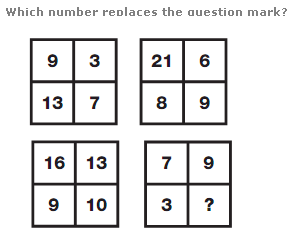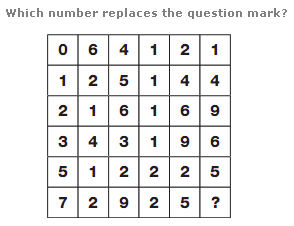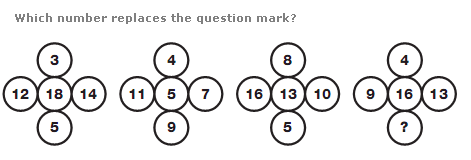# Puzzles - Number puzzles

Exercise : Number puzzles
166.:
5
Explanation
:
Add together numbers in corresponding segments of the outer four squares. The answer is placed in the diagonally opposite segment of the middle square.

167.:
6
Explanation
:
Split the diagram into 2 halves vertically, each 3 columns wide. Numbers in the left hand half follow the sequence of cubed numbers, from 64 to 729, and numbers in the right hand half follow the sequence of squared numbers, from 121 to 256.

168.